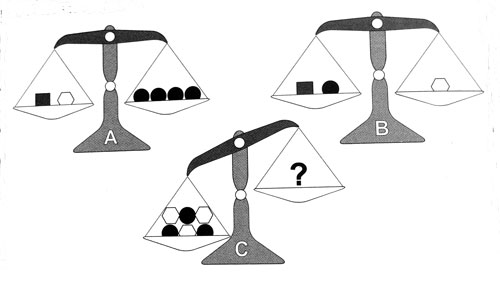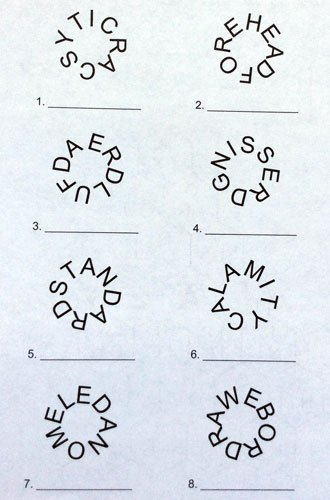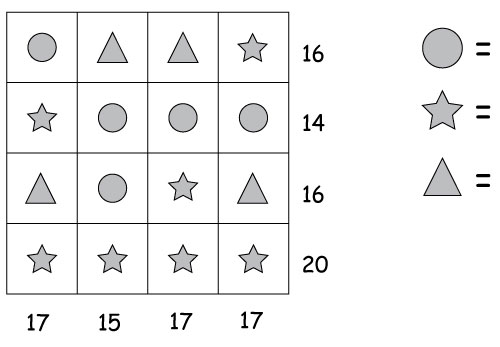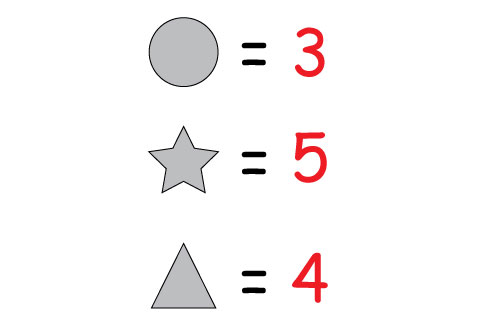Select Page

September 2015

How did you go?

Here are the answers to the September 2015 Brain Practice:

Balancing Puzzle

Scales A and B balance perfectly. How many squares will you need to balance scale C?

Two polygons weigh the same as 5 circles; 2 squares weigh the same as 3 circles and 3 polygons weigh the same as 5 squares, so 7 squares are needed.Word Circles

One word is contained in each circle – you can find it by reading either clockwise or anti-clockwise. Can you find them all?

1. Scarcity